# 项链 橙色传奇装备 - 火炬之光无限

icon affix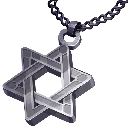流星

(100–120) 最大生命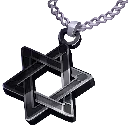幻影

(100–120) 最大魔力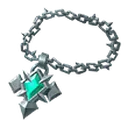抗争铁链

(20–30) 最大生命
(10–15)% 攻击与施法速度无主

(30–40) 最大生命
(8–12)% 攻击与施法速度
(20–30)% 投射物速度
+10% 移动速度
(15–20) 全属性磐岩的汪洋

(60–80) 最大生命
(60–80) 最大护盾
(20–25) 全属性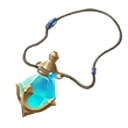灵佑之坠

(15–20) 全属性
(15–20)% 最大护盾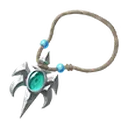塞壬的盲目

(60–80) 最大生命
(20–30)% 闪电抗性
(20–26)% 腐蚀抗性荒芜结晶

(15–20)% 最大生命
(40–60)% 物理伤害
(1500–2000) 护甲值防御宗师吊坠

(40–60) 最大生命
(120–150)% 从盾牌获得的防御值
(8–12)% 攻击格挡率
(10–15)% 元素抗性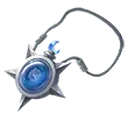厄运怀表

(60–70) 最大生命
(30–35) 最大护盾
(-20–-10)% 元素抗性
(200–300) 攻击和法术暴击值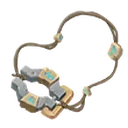贤者的铁枷

(15–20) 全属性
+20% 冷却回复速度

(60–80)% 魔力自然回复速度
(10–20)% 护盾充能速度漆黑月石项链

+1 防护技能等级
(44–52) 智慧洞明

(80–90) 最大生命

+40% 连续攻击几率
(20–30) 点力量与敏捷伪神之蜕

(12–15)% 全属性
<随机一条属性增益词缀>
<随机一条属性增益词缀>
<随机一条属性增益词缀>
<随机一条属性增益词缀>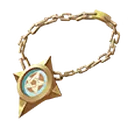五芒星盘

<随机一条攻击或法术物理点伤>
<随机一条攻击或法术火焰点伤>
<随机一条攻击或法术冰冷点伤>
<随机一条攻击或法术闪电点伤>
<随机一条攻击或法术腐蚀点伤>淬火之鸟的颈链

(10–15)% 最大生命和最大护盾
(10–15)% 元素抗性操幕之链

(10–15)% 最大护盾
(30–40) 点敏捷与智慧
(24–28)% 腐蚀抗性马内拉之雾

+1 腐蚀技能等级
(15–20) 全属性

(15–20)% 凋零伤害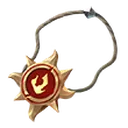落日涟漪

(50–80) 最大生命
+1 核心技能等级旋流之心

(30–40)% 暴击伤害
(40–50)% 暴击值
(-50–-40)% 技能范围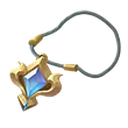真理

+1 全部技能等级
(55–65) 力量
(55–65) 敏捷
(55–65) 智慧
+250 反射腐蚀伤害畸雪

(50–70) 最大生命

(70–90)% 加剧效果
(24–28)% 冰冷抗性空弹之凶

(40–60) 最大生命
(10–20)% 投射物伤害

(-0.1–0.1) 秒装填时间
(10–30)% 闪电抗性神谕澄空

(-1–1) 聚能祝福层数上限

(8–10)% 元素抗性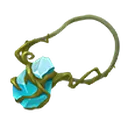魔泉之石

(15–20)% 最大魔力
(80–100) 最大魔力
+60% 魔力自然回复速度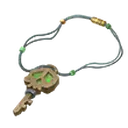典狱官的胸针

(40–50) 智慧
(20–30)% 召唤物最大生命
(40–50)% 召唤物伤害机巧魔盒猩红恶兆

(80–100) 最大生命
(12–16)% 最大生命
(12–16)% 元素抗性
+25% 几率造成异常状态幻海缠丝-血雨

(15–20)% 最大生命、最大魔力和最大护盾

-16% 生命自然回复速度

(30–50)% 受到的腐蚀伤害转化为物理伤害幻海缠丝-劫灰

(15–20)% 最大生命、最大魔力和最大护盾

-40% 火焰抗性
+1 坚韧祝福层数上限

(30–50)% 受到的腐蚀伤害转化为火焰伤害幻海缠丝-厉雷

(15–20)% 最大生命、最大魔力和最大护盾

-40% 闪电抗性
+1 灵动祝福层数上限

(30–50)% 受到的腐蚀伤害转化为闪电伤害幻海缠丝-苦寒

(15–20)% 最大生命、最大魔力和最大护盾

-40% 冰冷抗性
+1 聚能祝福层数上限

(30–50)% 受到的腐蚀伤害转化为冰冷伤害幻海缠丝-虚妄

(15–20)% 最大生命、最大魔力和最大护盾

(3–5)% 腐蚀抗性上限破碎日环

(60–80) 最大生命
(30–50)% 几率获得斩击次数

(20–30)% 火焰抗性
(800–1000) 护甲值永恒

(80–100) 最大生命玛格努斯的心火

(70–90) 最大生命
(6–10)% 力量
(35–45)% 火焰抗性
(8–12)% 火焰穿透母神围场

(20–30)% 防御值
(50–80)% 范围伤害

+2 地面蓄能层数上限暴怒之心

(60–100) 最大生命
(30–60) 力量

(60–90)% 暴击值银枪之语

(60–100) 最大生命
+30% 几率装填特殊弹药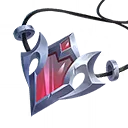虚空之镜

(100–120) 最大魔力战争号角

(-60–60)% 召唤物伤害

(-2–2) 智械召唤物最大数量上限
(-20–20)% 超载冷却回复速度